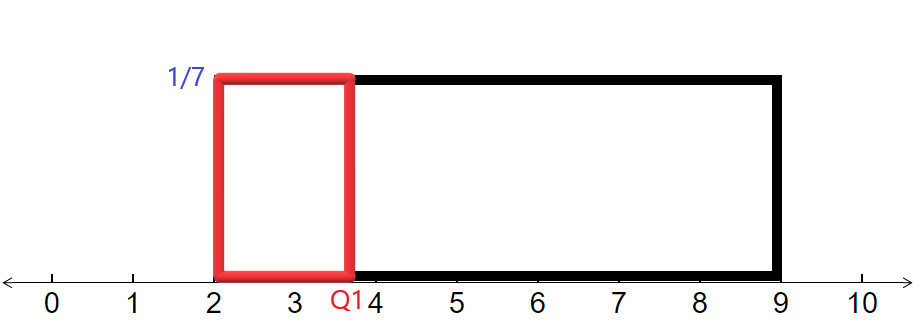$$\newcommand{\id}{\mathrm{id}}$$ $$\newcommand{\Span}{\mathrm{span}}$$ $$\newcommand{\kernel}{\mathrm{null}\,}$$ $$\newcommand{\range}{\mathrm{range}\,}$$ $$\newcommand{\RealPart}{\mathrm{Re}}$$ $$\newcommand{\ImaginaryPart}{\mathrm{Im}}$$ $$\newcommand{\Argument}{\mathrm{Arg}}$$ $$\newcommand{\norm}{\| #1 \|}$$ $$\newcommand{\inner}{\langle #1, #2 \rangle}$$ $$\newcommand{\Span}{\mathrm{span}}$$

3.1: Distance between Two Points on a Number Line

$$\newcommand{\vecs}{\overset { \rightharpoonup} {\mathbf{#1}} }$$

$$\newcommand{\vecd}{\overset{-\!-\!\rightharpoonup}{\vphantom{a}\smash {#1}}}$$

Learning Outcomes

1. Calculate the distance between two points on a number line when both are non-negative.
2. Calculate the distance between two points on a number line when at least one is negative.

The number line is the main visual base in statistics and we often want to look at two points on the number line and determine the distance between them. This is used to find the base of a rectangle or another figure that lies above the number line. By the end of this section, you will be able to determine the distance between any two points on a number line that comes from a statistics application.

Finding the Distance Between Two Points with Positive Coordinates on a Number Line

The key to finding the distance between two points is to remember that the geometric definition of subtraction is the distance between the two numbers as long as we subtract the smaller number from the larger.

Example $$\PageIndex{1}$$

Find the distance between the points 2.5 and 9.8 as shown below on the number line.Solution

To find the distance, we just subtract:

$9.8\:-\:2.5\:=\:7.3 \nonumber$

Example $$\PageIndex{2}$$

When finding probabilities involving a uniform distribution, we have to find the base of a rectangle that lies on a number line. Find the base of the rectangle shown below that represents a uniform distribution from 2 to 9.Solution

We just subtract:

$9\:-\:2\:=\:7 \nonumber$

Finding the Distance Between Two Points on a Number Line When the Coordinates Are Not Both Positive

In statistics, it is common to have points on a number line where the points are not both positive and we need to find the distance between them.

Example $$\PageIndex{3}$$

The diagram below shows the confidence interval for the difference between the proportion of men who are planning on going into the health care profession and the proportion of women. What is the width of the confidence interval?Solution

Whenever we want want to find the distance between two numbers, we always subtract. Recall that subtracting a negative number is adding.

$0.01\:-\:\left(-0.04\right)\:=\:0.01\:+\:0.04\:=\:0.05 \nonumber$

Therefore the width of the confidence interval is 0.05.

Example $$\PageIndex{4}$$

The mean value of credit card accounts is -6358 dollars. A study was done of recent college graduates and found their mean value for their credit card accounts was -5215 dollars. The number line below shows this situation. How far apart are these values?Solution

We subtract the two numbers and recall that when we subtract two negative numbers when we are looking at the right minus the left, we make them positive and subtract the positive numbers.

$-5215\:-\:\left(-6358\right)\:=\:6358\:-\:5215\:=\:1143 \nonumber$

Thus the mean credit card balances are \$1143 apart.

Exercise

In statistics, we are asked to find a z-score, which tells us how unusual an event is. The first step in finding a z-score is to calculate the distance a value is from the mean. The number line below depicts the mean of 18.56 and the value of 20.43. Find the distance between these two points.